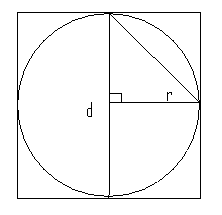# SAT Math: Circles Inscribed in Squares

In this series, we will cover many types of geometric scenarios encountered on the SAT Math test. A basic knowledge of simple formulas (area, perimeter, etc.) is essential, but there are numerous shortcuts to geometry questions that will save you time. Today, we’ll explore circles inscribed in squares.
Some Things to Remember• The center of the square is the same point as the center of the circle
• Draw lines! Depending on what the stimulus asks for, draw in lines that create simple shapes. (Squares can be turned into triangles, for example.)
• Shared angles will normally not be explicitly stated, unless necessary.
• Trust the pictures, but not too much. Inferences must be drawn from fact. Just because it looks like 90-degrees doesn’t mean it is! (Many of these common inferences will be detailed in this series.)
• Lengths cannot be negative. Be careful in DS questions that pose equations in the context of quadratic equations with two solutions. If one solution is negative and the other is positive, only the positive solution remains and the information is sufficient.

### For circles:

• d=2r and all lines from the center to the exterior equal r.
• C = 2πr = πd
• A = πr²
• NEVER use 2πr² unless you are adding the areas of identical circles!
• Tangent lines create right angles with the radius that meets that tangent.
• If you know r, you know everything about the circle!
• Use π = 22/7 with caution. Remember 22/7 > π.

### For squares:

• The diagonal equals s√2, since it creates 45-degree angles.
• The intersection of the diagonals creates a right angle.
• When a circle is inscribed inside a square, the side equals the diameter.

Usually, you will be provided with one bit of information that tells you a whole lot, if not everything. If given the length of the side of the square in the above image, we can actually find the length of the hypotenuse of the internal triangle (s = d = 2r, so the hypotenuse = (s√2)/2).

Find the large area and subtract the small area from it. When dealing with circles along with other figures, eliminate answer choices that ONLY have π’s in them or don’t have any at all. Typically, your answer will look like x + yπ.The picture above depicts a circle perfectly inscribed in a square. Is the shaded region > 4?
(1) The area of the large rectangle equals 64.
(2) The perimeter of the shaded region equals 8 + 2π.

Statement 1: By knowing the area of the large square we also know the lengths of its sides. (Note that 64 is a perfect square, which should be a clue.) If the side is 8, then so is the diameter, which means the radius equals 4. In the image, we can see that the “larger figure” is the top-right square bordered by two radii and the outer border. How do we know that it’s a square? Two pieces of information: All sides are equal to 4, and the radius meets the large square at a right angle because it is a tangent. In this instance, the area of the smaller square equals 16. Since the interior angle is 90-degrees (360/4), the area of the sector of the circle can be represented by A = πr²/4. So that A = 16π/4 = 4π.
A(shaded) = A(small square) – A(sector) = 16 – 4π < 4 because 4π > 12. Sufficient.
Statement 2: The first thing that should jump out is the combination of a π-term and non- π-term. We can reasonably assume that 8 represents the two straight sides of the perimeter and the 2π the arc-length of the quarter circle, which is known because of the internal right angle. If 2π = C/4, then C = 8π. If C = 8π, then r = 4. From here, we return to the same reasoning as above:
A(shaded) = A(small square) – A(sector) = 16 – 4π < 4 because 4π > 12. Sufficient.
Each statement is sufficient, so the answer is choice D.

### Two important takeaways:

1. Never assume without proof.